Midterm 2 Preparation

# Midterm 2: Version A

Find the solution set of the system graphically.

1.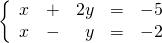For problems 2–4, find the solution set of each system by any convenient method.

1.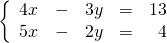2.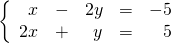3.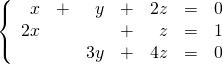Reduce the following expressions in questions 5–7.

1.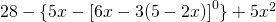2.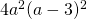3.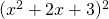Divide using long division.

1.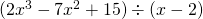For problems 9–12, factor each expression completely.

1.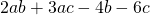2.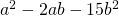3.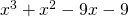4.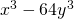Solve the following word problems.

1. The sum of a brother’s and sister’s ages is 35. Ten years ago, the brother was twice his sister’s age. How old are they now?
2. Kyra gave her brother Mark a logic question to solve: If she has 20 coins in her pocket worth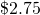, and if the coins are only dimes and quarters, how many of each kind of coin does she have?
3. A 50 kg blend of two different grades of tea is sold for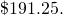If grade A sells for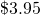per kg and grade B sells for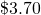per kg, how many kg of each grade were used?

<a class=”internal” href=”/intermediatealgebraberg/back-matter/midterm-two-version-a-answer-key/”>Midterm 2: Version A Answer Key